PRINTABLE FOR KIDS

XII (12) HSC

XI (11) FYJC
X (10) SSC

### Practice Set 4.4 Financial Planning Class 10th Mathematics Part 1 MHB Solution

Practice Set 4.4

1. Market value of a share is Rs. 200. If the brokerage rate is 0.3% then find the…
2. A share is sold for the market value of Rs. 1000. Brokerage is paid at the rate of…
3. Fill in the blanks given in the contract note of sale-purchase of shares. (B - buy S -…
4. Smt. Desai sold shares of face value Rs. 100 when the market value was Rs. 50 and…
5. Mr. D'souza purchased 200 shares of FV Rs. 50 at a premium of Rs. 100. He received 50%…

###### Practice Set 4.4

Question 1.

Market value of a share is Rs. 200. If the brokerage rate is 0.3% then find the purchase value of the share.

Given, MV = Rs. 200

Brokerage rate = 0.3%

We know that purchase value of a share = MV + Brokerage

∴ Purchase value of a share = 200 + 0.3% of 200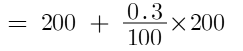= 200 + 0.60

= Rs. 200.60

Question 2.

A share is sold for the market value of Rs. 1000. Brokerage is paid at the rate of 0.1%. What is the amount received after the sale?

Given, MV = Rs. 1000

Brokerage rate = 0.1%

We know that the selling price for a share = MV – Brokerage rate

∴ Selling price for a share = 1000 – 0.1% of 1000

= 1000 – 1

= 999

Question 3.

Fill in the blanks given in the contract note of sale-purchase of shares.

(B - buy S - sell)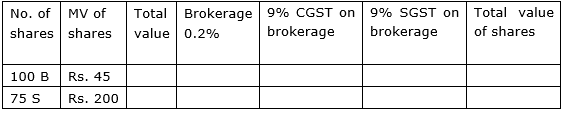We know that Investment (total value) = Number of shares × MV

∴ Total value = 100 × 45

= Rs. 4500

We know that brokerage = Total Value × Brokerage rate

∴ Brokerage = 4500 × 0.2%

= Rs. 9

We know that CGST on brokerage = brokerage × CGST rate

∴ CGST on brokerage = 9 × 9%

= Rs. 0.81

We know that CGST value is equal to SGST value.

∴ SGST on brokerage = Rs. 0.81

We know that total value of shares = Total Value + Brokerage + CGST on brokerage + SGST on brokerage

⇒ Total value of shares = 4500 + 9 + 0.81 + 0.81

= Rs. 4510.62

S-Sell:

Total value = 200 × 75

= Rs. 15000

Brokerage = 15000 × 0.2%

= Rs. 30

CGST on brokerage = 30 × 9%

= Rs. 2.70 = SGST on brokerage

Total value of shares = 15000 + 30 + 2.70 + 2.70

= Rs. 14964.60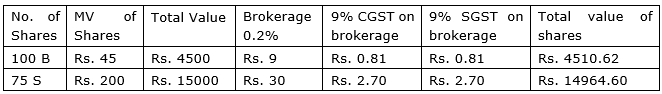Question 4.

Smt. Desai sold shares of face value Rs. 100 when the market value was Rs. 50 and received Rs. 4988.20. She paid brokerage 0.2% and GST on brokerage 18%, then how many shares did she sell?

Given, FV = Rs. 100

MV = Rs. 50

Total Value of shares = Rs. 4988.20

Brokerage 0.2% =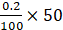= Rs. 0.1

GST per share on Brokerage = 18% of 0.1 = 0.018

We know that cost of a share = MV + Brokerage + GST

∴ Cost of 1 share = 50 + 0.1 + 0.018 = Rs. 50.118

We know that total value of shares = Total Value + Brokerage + GST on brokerage

∴ 4988.20 = Total Value + 0.1 + 0.018

⇒ Total Value = 4988.20 – 0.1 – 0.018

∴ Total Value = Rs. 4988.082

We know that Investment (Total Value) = Cost × Number of shares

∴ Number of shares =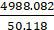= 99.5 ≈ 100

Question 5.

Mr. D'souza purchased 200 shares of FV Rs. 50 at a premium of Rs. 100. He received 50% dividend on the shares. After receiving the dividend he sold 100 shares at a discount of Rs. 10 and remaining shares were sold at a premium of Rs. 75. For each trade he paid the brokerage of Rs. 20. Find whether Mr. D'souza gained or incurred a loss? by how much?

Given:

Number of shares Mr. D’souza purchased = 200

FV = Rs. 50

Premium = Rs. 100

We know that if MV > FV, then the share is at premium.

∴ MV = FV + Premium

⇒ MV = 50 + 100 = Rs. 150

Value of 200 shares = 200 × 150 = Rs 30,000

We know that dividend per share =

∴ Dividend per share =

= Rs. 25

∴ Total dividend received = 200 × 25 = Rs. 5000

100 shares sold at discount of Rs 10.

∴ Selling price of 100 shares = 100 × (50-10)

= 100 × 40

= Rs 4000

Amount received on selling 100 shares = selling price – brokerage

= 4000 – 20

= Rs 3980

Another 100 shares were sold at a premium of Rs. 75.

∴ MV = FV + Premium

⇒ MV = 50 + 75 = Rs. 125

Selling price of 100 shares = 100× 125

= Rs 12500

Amount received on selling 100 shares = selling price – brokerage

= 12500 – 20

= Rs 12480

Total amount = 5000 + 3980 + 12480

= Rs 21460

Investment = 30,000

Loss = Investment – amount on selling the shares

= 30,000 – 21460

= Rs 8560

## PDF FILE TO YOUR EMAIL IMMEDIATELY PURCHASE NOTES & PAPER SOLUTION. @ Rs. 50/- each (GST extra)

SUBJECTS

HINDI ENTIRE PAPER SOLUTION

MARATHI PAPER SOLUTION
SSC MATHS I PAPER SOLUTION
SSC MATHS II PAPER SOLUTION
SSC SCIENCE I PAPER SOLUTION
SSC SCIENCE II PAPER SOLUTION
SSC ENGLISH PAPER SOLUTION
SSC & HSC ENGLISH WRITING SKILL
HSC ACCOUNTS NOTES
HSC OCM NOTES
HSC ECONOMICS NOTES
HSC SECRETARIAL PRACTICE NOTES

2019 Board Paper Solution

HSC ENGLISH SET A 2019 21st February, 2019

HSC ENGLISH SET B 2019 21st February, 2019

HSC ENGLISH SET C 2019 21st February, 2019

HSC ENGLISH SET D 2019 21st February, 2019

SECRETARIAL PRACTICE (S.P) 2019 25th February, 2019

HSC XII PHYSICS 2019 25th February, 2019

CHEMISTRY XII HSC SOLUTION 27th, February, 2019

OCM PAPER SOLUTION 2019 27th, February, 2019

HSC MATHS PAPER SOLUTION COMMERCE, 2nd March, 2019

HSC MATHS PAPER SOLUTION SCIENCE 2nd, March, 2019

SSC ENGLISH STD 10 5TH MARCH, 2019.

HSC XII ACCOUNTS 2019 6th March, 2019

HSC XII BIOLOGY 2019 6TH March, 2019

HSC XII ECONOMICS 9Th March 2019

SSC Maths I March 2019 Solution 10th Standard11th, March, 2019

SSC MATHS II MARCH 2019 SOLUTION 10TH STD.13th March, 2019

SSC SCIENCE I MARCH 2019 SOLUTION 10TH STD. 15th March, 2019.

SSC SCIENCE II MARCH 2019 SOLUTION 10TH STD. 18th March, 2019.

SSC SOCIAL SCIENCE I MARCH 2019 SOLUTION20th March, 2019

SSC SOCIAL SCIENCE II MARCH 2019 SOLUTION, 22nd March, 2019

XII CBSE - BOARD - MARCH - 2019 ENGLISH - QP + SOLUTIONS, 2nd March, 2019

HSC Maharashtra Board Papers 2020

(Std 12th English Medium)

HSC ECONOMICS MARCH 2020

HSC OCM MARCH 2020

HSC ACCOUNTS MARCH 2020

HSC S.P. MARCH 2020

HSC ENGLISH MARCH 2020

HSC HINDI MARCH 2020

HSC MARATHI MARCH 2020

HSC MATHS MARCH 2020

SSC Maharashtra Board Papers 2020

(Std 10th English Medium)

English MARCH 2020

HindI MARCH 2020

Hindi (Composite) MARCH 2020

Marathi MARCH 2020

Mathematics (Paper 1) MARCH 2020

Mathematics (Paper 2) MARCH 2020

Sanskrit MARCH 2020

Important-formula

THANKS